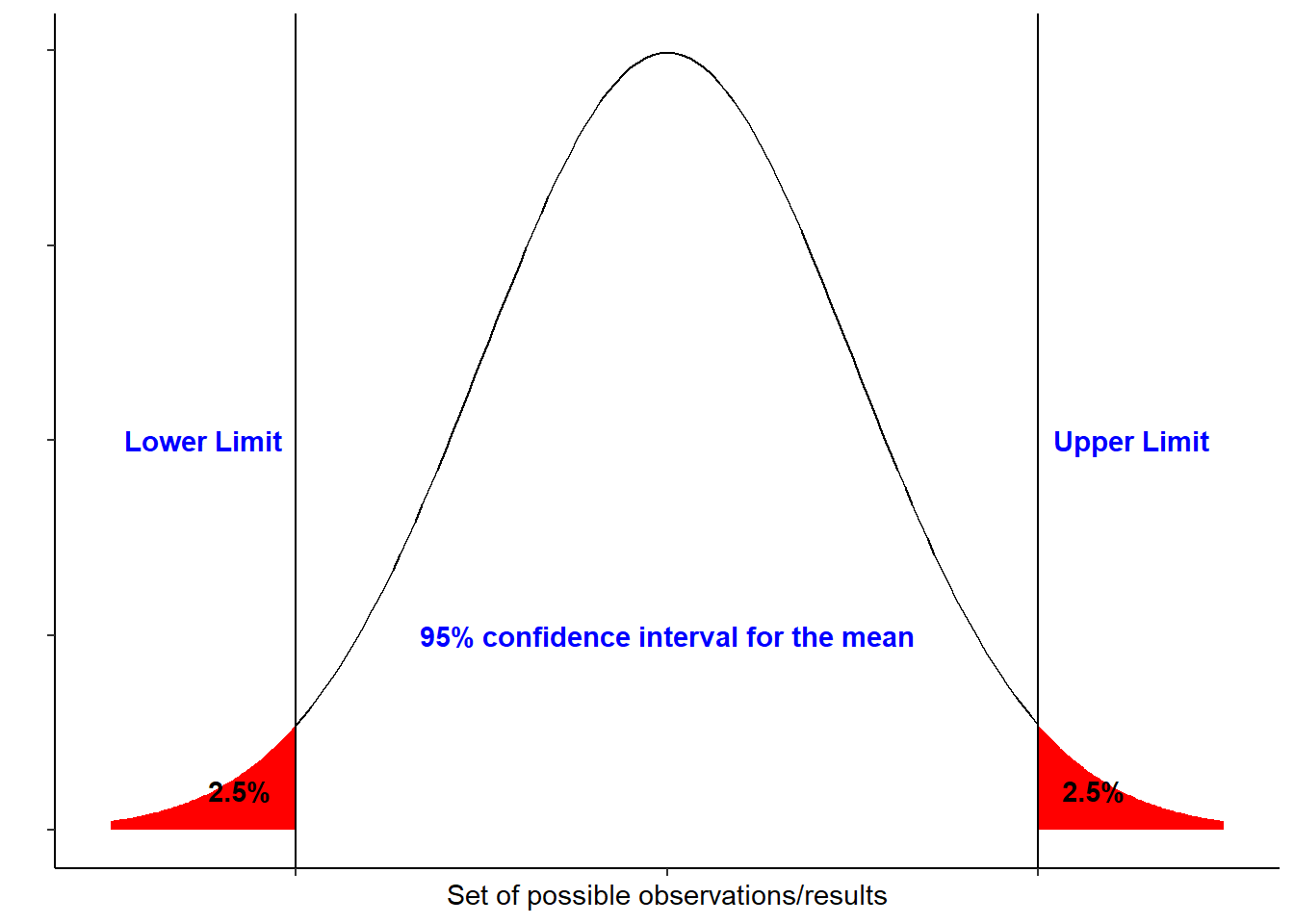# Articles by Home

### Calculating Confidence Interval in R

April 15, 2021 |

Confidence intervals (CI) are part of inferential statistics that help in making inference about a population from a sample. Based on the confidence level, a true population mean is likely covered by a range of values called confidence interval. A ba...### Tutorial – make population pyramids with Queensland data

April 13, 2018 |

In this tutorial I will use Queensland population data already downloaded from the Australian Bureau of Statistics (ABS), and rearrange the data to produce population pyramids using ggplot. The population pyramid is one of the most popular methods to visualise population age structure. The method constructs a chart with each ... [Read more...]

### An R tutorial to download and plot some Queensland population data

April 12, 2018 |

Introduction In this tutorial I will download Queensland population data from the Australian Bureau of Statistics (ABS), tidy it up a bit, and plot some graphs with ggplot. The data in the spreadsheet are not in the most useful format so this tutorial will use tidyverse tools to manipulate the ... [Read more...]